# The Futures Pricing

View chapters →

## 10.1 – The Pricing Formula

If you were to take a conventional course on Futures trading, you would probably be introduced to the futures pricing formula right at the very beginning of the course. However we have deliberately opted to talk about it now, at a much later stage. The reason is simple – if you are trading futures based on technical analysis (I assume a vast majority of you are doing this) then you would not really need to know how the futures are priced, although a good working knowledge would help. However if you aspire to trade futures by employing quantitative strategies such as Calendar Spreads or Index Arbitrage then you certainly need to know this. In fact we will have a module dedicated to ‘Trading Strategies’ where we would discuss some of these strategies, hence the discussion in this chapter will lay down a foundation for the forthcoming modules.

If you recall, in some of the earlier chapters occasionally we discussed the ‘Futures Pricing Formula’ as the prime reason for the difference between the spot price and the futures price. Well, I guess it is time now to lift the veil and introduce the ‘Future Pricing Formula’.

We know the futures instrument derives its value from its respective underlying. We also know that the futures instrument moves in sync with its underlying. If the underlying price falls, so would the futures price and vice versa. However, the underlying price and the futures price differs and they are not really the same. To give you a perspective as I write this, Nifty Spot is at 8,845.5 whereas the corresponding current month contract is trading at 8,854.7, please refer to the snap shot below. This difference in price between the futures price and the spot price is called the “basis or spread”. In case of the Nifty example below, the spread is 9.2 points (8854.7 – 8845.5).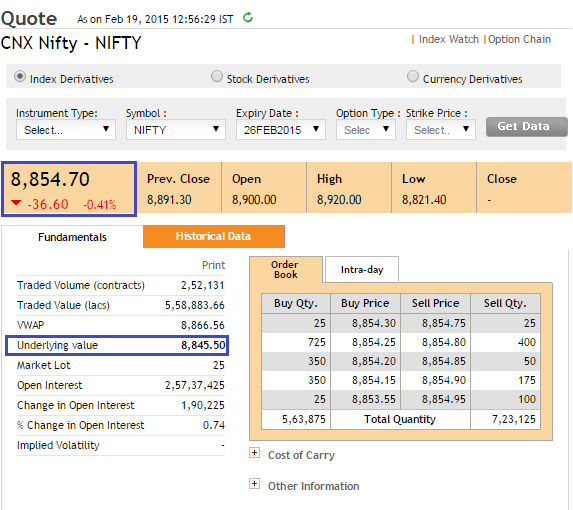The difference in price is attributable to the ‘Spot – Future Parity’. The spot future parity the difference between the spot and futures price that arises due to variables such as interest rates, dividends, time to expiry etc. In a very loose sense it is simply is a mathematical expression to equate the underlying price and its corresponding futures price. This is also known as the futures pricing formula.

The futures pricing formula simply states –

Futures Price = Spot price *(1+ rf )– d

Where,

rf = Risk-free rate

d – Dividend

Note, ‘rf’ is the risk-free rate that you can earn for the entire year (365 days); considering the expiry is at 1, 2, and 3 months one may want to scale it proportionately for time periods other than the exact 365 days. Therefore a more generic formula would be –

Futures Price = Spot price * [1+ rf*(x/365)]– d

Where,

x = number of days to expiry.

One can take the RBI’s 91 day Treasury bill as a proxy for the short term risk-free rate. You can find the same on the RBI’s home page, as shown in the snapshot below –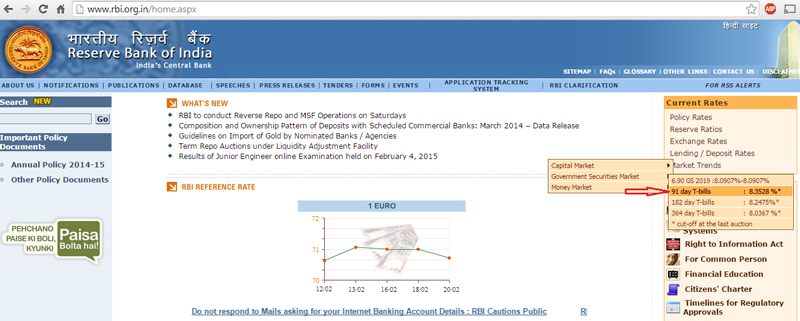As we can see from the image above, the current rate is 8.3528%. Keeping this in perspective let us work on a pricing example. Assume Infosys spot is trading at 2,280.5 with 7 more days to expiry, what should Infosys’s current month futures contract be priced at?

Futures Price = 2280.5 * [1+8.3528 %( 7/365)] – 0

Do note, Infosys is not expected to pay any dividend over the next 7 days, hence I have assumed dividend as 0. Solving the above equation, the future price turns out to be 2283. This is called the ‘Fair value’ of futures. However the actual futures price as you can see from the image below is 2284. The actual price at which the futures contract trades is called the ‘Market Price’.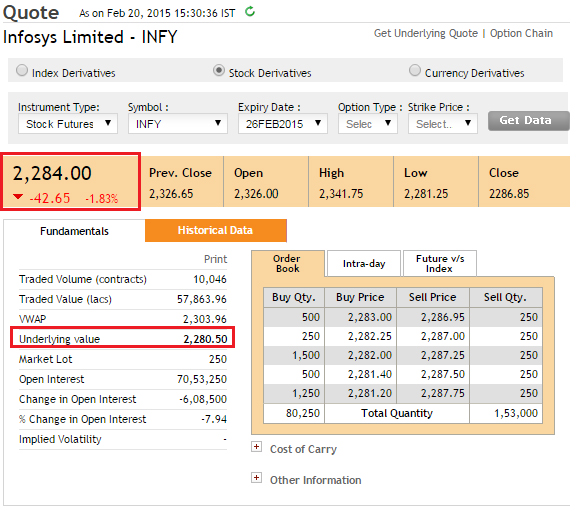The difference between the fair value and market price mainly occurs due to market costs such as transaction charges, taxes, margins etc. However by and large the fair value reflects where the futures should be trading at a given risk free rate and number of days to expiry. Let us take this further, and figure out the futures price for mid month and far month contracts.

Mid month calculation

Number of days to expiry = 34 (as the contract expires on 26th March 2015)

Futures Price = 2280.5 * [1+8.3528 %( 34/365)] – 0

= 2299

Far month calculation

Number of days to expiry = 80 (as the contract expires on 30th April 2015)

Futures Price = 2280.5 * [1+8.3528 %( 80/365)] – 0

= 2322

From NSE website let us take a look at the actual market prices –

Snapshot of Infosys’s mid month contract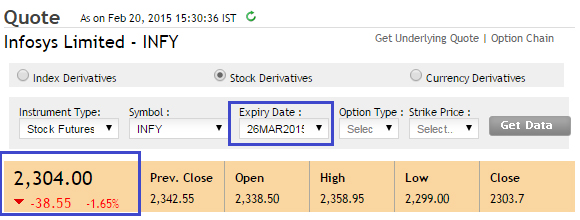Snapshot of Infosys’s mid month contractClearly there is a difference between the calculated fair value and the market price. I would attribute this to the applicable costs. Besides, the market could be factoring in some financial yearend dividends as well. However the key point to note is as the number of days to expiry increases, the difference between the fair value and market value widens.

In fact this leads us to another important commonly used market terminology – the discount and the premium.

If the futures is trading higher than the spot, which mathematically speaking is the natural order of things, then the futures market is said to be at ‘premium’. While ‘Premium’ is a term used in the Equity derivatives markets, the commodity derivatives market prefer to refer to the same phenomenon as ‘Contango’. However, both contango and premium refer to the same fact – The Futures are trading higher than the Spot.

Here is a plot of Nifty spot and its corresponding futures for the January 2015 series. As you can see the Nifty futures is trading above the spot during the entire series.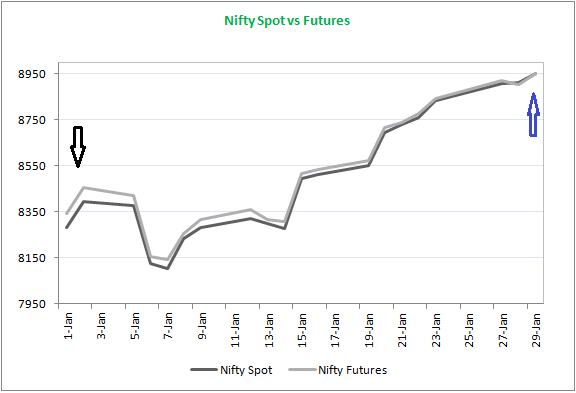I specifically want to draw your attention to the following few points –

1. At the start of the series (highlighted by a black arrow) the spread between the spot and futures is quite high. This is because the number of days to expiry is high hence the x/365 factor in the futures pricing formula is also high.
2. The futures remained at premium to the spot throughout the series
3. At the end of the series (highlighted by a blue arrow) the futures and the spot have converged. In fact this always happens. Irrespective of whether the future is at a premium or a discount, on the day of the expiry, the futures and spot will always converge.
4. If you have a futures position and if you fail to square off the position by expiry, then the exchange will square off the position automatically and it will be settled at the spot price as both futures and spot converges on the day of the expiry

Not always does the futures trade richer than the spot. There could be instances – mainly owing to short term demand and supply imbalances where the futures would trade cheaper than its corresponding spot. This situation is when the futures is said to be trading at a discount to the spot. In the commodities world, the same situation is referred to as the “backwardation”.

## 10.2 – Practical Application

Before we conclude this chapter, let us put the futures pricing formula to some practical use. Like I had mentioned earlier, futures pricing formula comes very handy when you aspire to trade employing quantitative trading techniques. Please note, the following discussion is only a preview window into the world of trading strategies. We will discuss all these things plus more in greater detail when we take up the module on “Trading Strategies”. Consider this situation –

Wipro Spot = 653

Rf – 8.35%

x = 30

d = 0

Given this, the futures should be trading at –

Futures Price = 653*(1+8.35 %( 30/365)) – 0

= 658

Accommodate for market charges, the futures should be trading in and around 658. Now what if instead the futures contract is trading at a drastically different price? Let’s say 700? Clearly there is a trade here. The difference between the spot and futures should ideally be just 5 points, but due to market imbalances the difference has shot up to 47 points. This is a spread that we can capture by deploying a trade.

Here is how one can do this – since the future contract is trading above its fair value, we term the futures market price as expensive relative to its fair value. Alternatively we can say, the spot is trading cheaper with respect to the futures.

The thumb rule in any sort of ‘spread trade’ is to buy the cheaper asset and sell the expensive one. Hence going by this, we can sell Wipro Futures on one hand and simultaneously buy Wipro in the spot market. Let us plug in the numbers and see how this goes –

Buy Wipro in Spot @ 653

Sell Wipro in Futures @ 700

Now we know that on the expiry day, both the spot and the futures converge into one single price (refer to the Nifty graph posted above). Let us assume a few random values at which the futures and the spot converge – 675, 645, 715 and identify what happens to the trade –

Expiry Value Spot Trade P&L (Long) Futures Trade P&L (Short) Net P&L
675 675 – 653 = +22 700 – 675 = +25 +22 + 25 = +47
645 645 – 653 = -08 700 – 645 = +55 -08 + 55 = +47
715 715 – 653 = +62 700 – 715 = -15 +62 – 15 = +47

As you can notice, once you have executed the trade at the expected price you have essentially locked in the spread. So irrespective of where the market goes by expiry, the profits are guaranteed! Of course, it goes without saying that it makes sense to square off the positions just before the expiry of the futures contract. This would require you to sell Wipro in spot market and buy back Wipro in Futures market.

This kind of trade between the futures and the spot to extract and profit from the spread is also called the ‘Cash & Carry Arbitrage’.

The calendar spread is a simple extension of the cash & carry arbitrage. In a calendar spread, we attempt to extract and profit from the spread created between two futures contracts of the same underlying but with different expiries. Let us continue with the Wipro example and understand this better –

Wipro Spot is trading at = 653

Current month futures fair value (30 days to expiry) = 658

Actual market value of current month futures = 700

Mid month futures fair value (65 days to expiry) = 663

Actual market value of mid month futures = 665

From the above example, clearly the current month futures contract is trading way above its expected theoretical fair value. However the mid month contract is trading close to its actual fair value estimate. With these observations, I will make an assumption that the current month contract’s basis will eventually narrow down and the mid month contract will continue to trade close to its fair value.

Now with respect to the mid month contract, the current month contract appears to be expensive. Hence we sell the expensive contract and buy the relatively cheaper one. Therefore the trade set up would require me to buy the mid month futures contract @ 665 and sell the current month contract @ 700.

What do you think is the spread here? Well, the spread is the difference between the two future contracts i.e 700 – 665 = 35 points.

The trade set up to capture the spread goes like this –

Sell the current month futures @ 700

Buy the mid month futures @ 665

Do note – because you are buying and selling the same underlying futures of different expiries, the margins are greatly reduced as this is a hedged position.

Now after initiating the trade, one has to wait for the current month’s futures to expire.  Upon expiry, we know the current month futures and the spot will converge to a single price. Of course on a more practical note, it makes sense to unwind the trade just before the expiry.

Let us arbitrarily take a few scenarios as below and see how the P&L pans out –

Expiry Value Current month P&L (Short) Mid Month P&L (Long) Net P&L
660 700 – 660 = +40 660 – 665 = -5 +40 – 5 = +35
690 700 – 690 = +10 690 – 665 = +25 +10 + 25 = +35
725 700 – 725 = -25 725 – 665 = +60 -25 + 60 = +35

Of course, do recall the critical assumption we have made here is that i.e. the mid month contract will stick close to its fair value. From my trading experience this happens most of the times.

Most importantly please do bear in mind the discussion with respect to spreads in this chapter is just a sneak peek into the world of trading strategies. We will discuss these strategies in a separate module which would give you an in depth analysis on how one can professionally deploy these strategies.

### Key takeaways from this chapter

1. The futures pricing formula states that the Futures Price = Spot price *(1+Rf (x/365)) – d
2. The difference between futures and spot is called the basis or simply the spread
3. The futures price as estimated by the pricing formula is called the “Theoretical fair value”
4. The price at which the futures trade in the market is called the ‘market value’
5. The theoretical fair value of futures and market value by and large should be around the same value. However there could be slight variance mainly due to the associated costs
6. If the futures is rich to spot then the futures is said to be at premium else it is said to be at a discount
7. In commodity parlance Premium = Contango and Discount = Backwardation
8. Cash and carry is a spread where one can buy in the spot and sell in the futures
9. Calendar spread is an extension of a cash and carry where one buys a contract and simultaneously sells another contract (with a different expiry) but of the same underlying

1.amit pathak says:

Hi karthik,
Pls explain this : As the number of days to expiry increases, the difference between the fair value and market value widens.

•Karthik Rangappa says:

The x/365 factor, where x represents number of days increases when x is large. For example when there are 30 days to expiry, x/365 is 0.082, however when when x is 20, x/365 is 0.54. This makes the spread to increase.

•amit pathak says:

At times during the contract cycle commentators say premium in nifty futures has increased,inspite of the fact that time is diminishing with every passing day of the contract.Accordingly they analyse future prospects.Pls help me understand this.

•Karthik Rangappa says:

Besides time other factors also contribute. This is mainly due to the demand and supply in the contract.

•amit pathak says:

Hi Karthik,
what impact volatility will have on premium of nifty futures ?

•Karthik Rangappa says:

Nice question. As far as I know volatility and futures premium are not directly related (at least in their mathematical equation). I guess this is because futures is a linear instrument where the only factor that affects its movement is “direction”. On the other hand options premium tends to shoot up when volatility increases. More on this topic when we take up options theory.

•skburman26 says:

Hello Karthik, a doubt.
The price of stock future is always more than its underlying asset, the equity price.
And the prices converge when the future is about to expire.
So when a stock future is priced lower than its equity counterpart, what does it imply?

•Karthik Rangappa says:

It means the futures are trading at a discount to spot. Discount situation comes about when futures are heavily sold in the market. All else equal, futures are always at a premium to spot.

•ARUN says:

correction req :: when when x is 20, x/365 should be 0.054.

•Karthik Rangappa says:

Thanks for pointing this, let me look into this.

•Madhukar says:

@nitin
In fair value calculation of nifty future is it necessary to consider dividends of underlying stocks!

•Karthik Rangappa says:

DCF does not really take dividend into consideration, Madhukar.

2.NARSIMHA says:

sir,is there any stategy r software to find profitable c spreads in futures,&should we close all positions at atime r can we take advantage of one fut according to mkt&should we wait till expiry,will it not be too long what if get profit of 2000/trade daily r swing should we square off r wait.in this way cant we make 25 trades/month clarify

•Karthik Rangappa says:

Based on our earlier interaction, I would suggest you stick to simple swing trade for now before you venture into calender spreads.

3.NARSIMHA says:

sir,evrytime when taking positions in calender spreads(2 futures)should we have to calculate fair value r is there any thumb rule to calculatein %terms for near&mid month explain

•Karthik Rangappa says:

There is a workaround for this, which I will explain in the “Trading Strategies” module.

4.Saurabh says:

Hi Karthik,

One question that comes to my mind is that how to find such trader? Can we automate a strategy on pi that automatically searches for scrips that have the C&C arbitrage or normal spread? Similarly, if we were to calculate the fair price for each and every scrip then the trade opportunity would be lost.

Thoughts?

•Karthik Rangappa says:

There is a different quant angle to this Saurabh. I will share it shortly.

•Naresh says:

ARBITRAGE – Hi ! If futures for near month is available on discount, then can one buy 2 ATM puts and go long futures in order to benefit from the same spread ? Is it worth it knowing that the profitability may be low ! Thanks

•Karthik Rangappa says:

Sounds interesting, but honestly you need to back test this before diving in. Chances are that the discount may persist through the expiry week and spot may come down to futures on expiry.

5.madhu nair says:

hi karthik, can the last candle be traded as a bullish harami? do the indicators suggest a long trade?

•Karthik Rangappa says:

Just by candlestick perspective it does, in fact its perfect. However I would not get carried away with it, I would check for other checklist items and confirm if it matches.Good luck.

15 chapters

22 chapters

16 chapters

13 chapters

25 chapters

14 chapters

7 chapters

19 chapters

16 chapters

16 chapters

32 chapters

603 chapters

18 chapters

9 chapters